Ex 12.1

Chapter 12 Class 9 Statistics
Serial order wise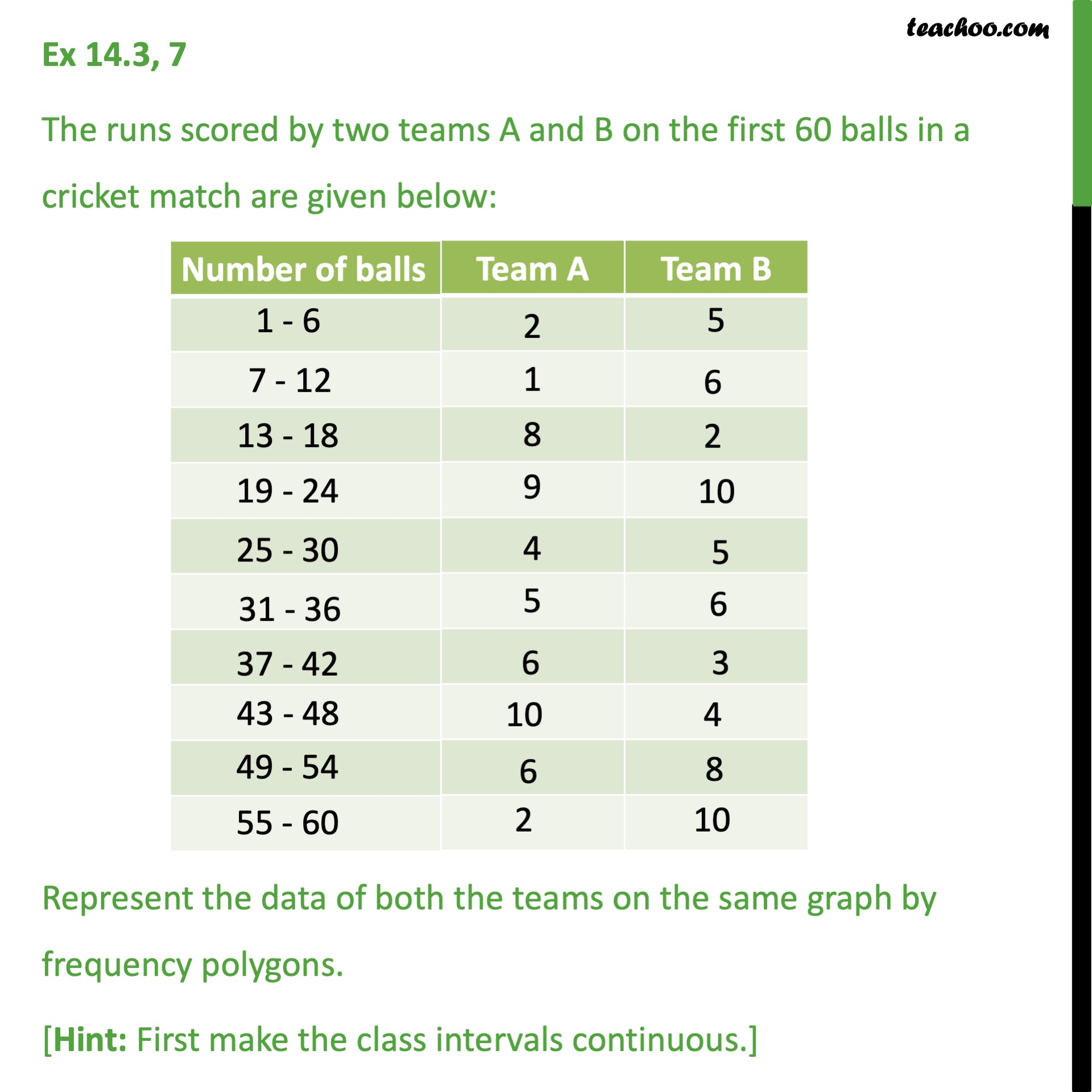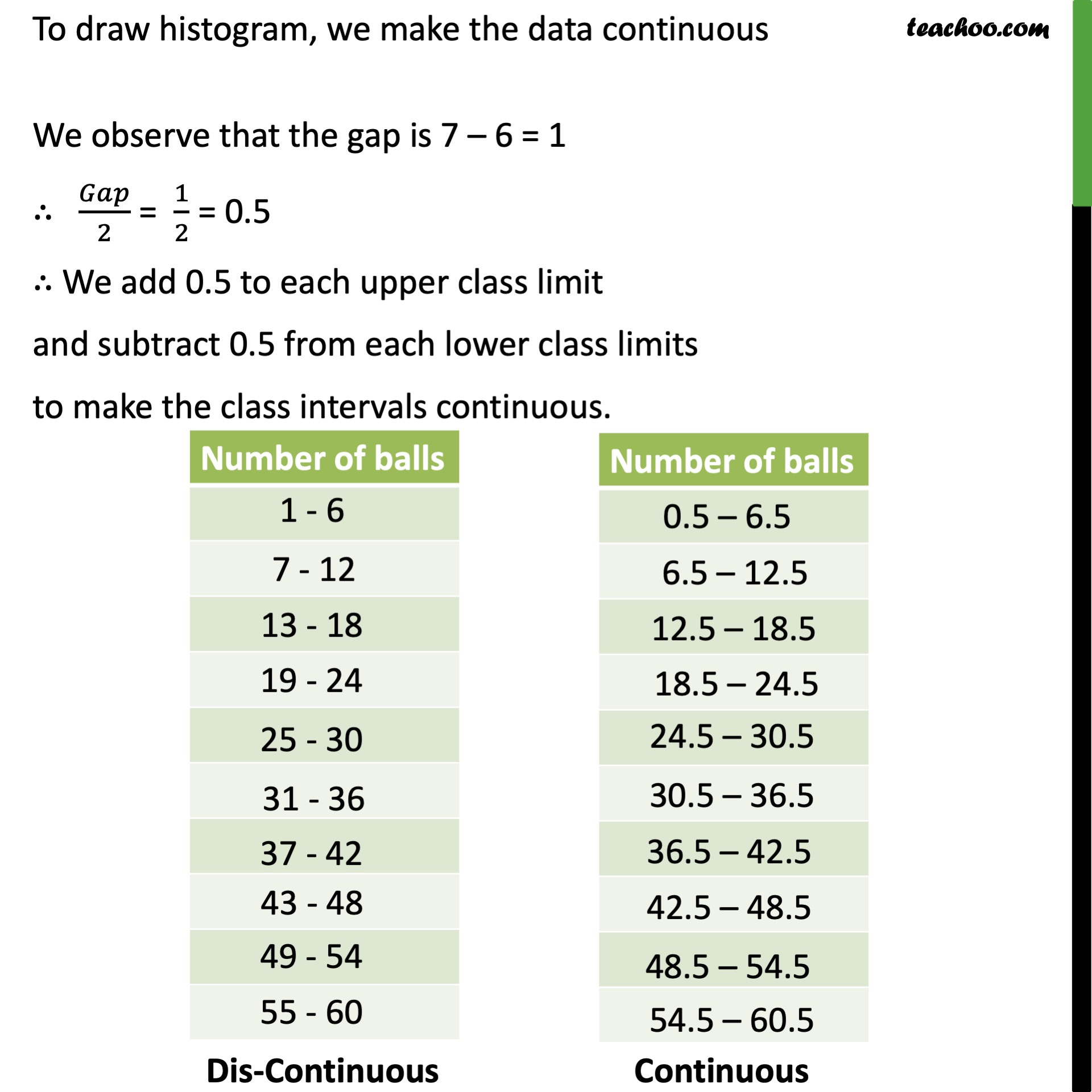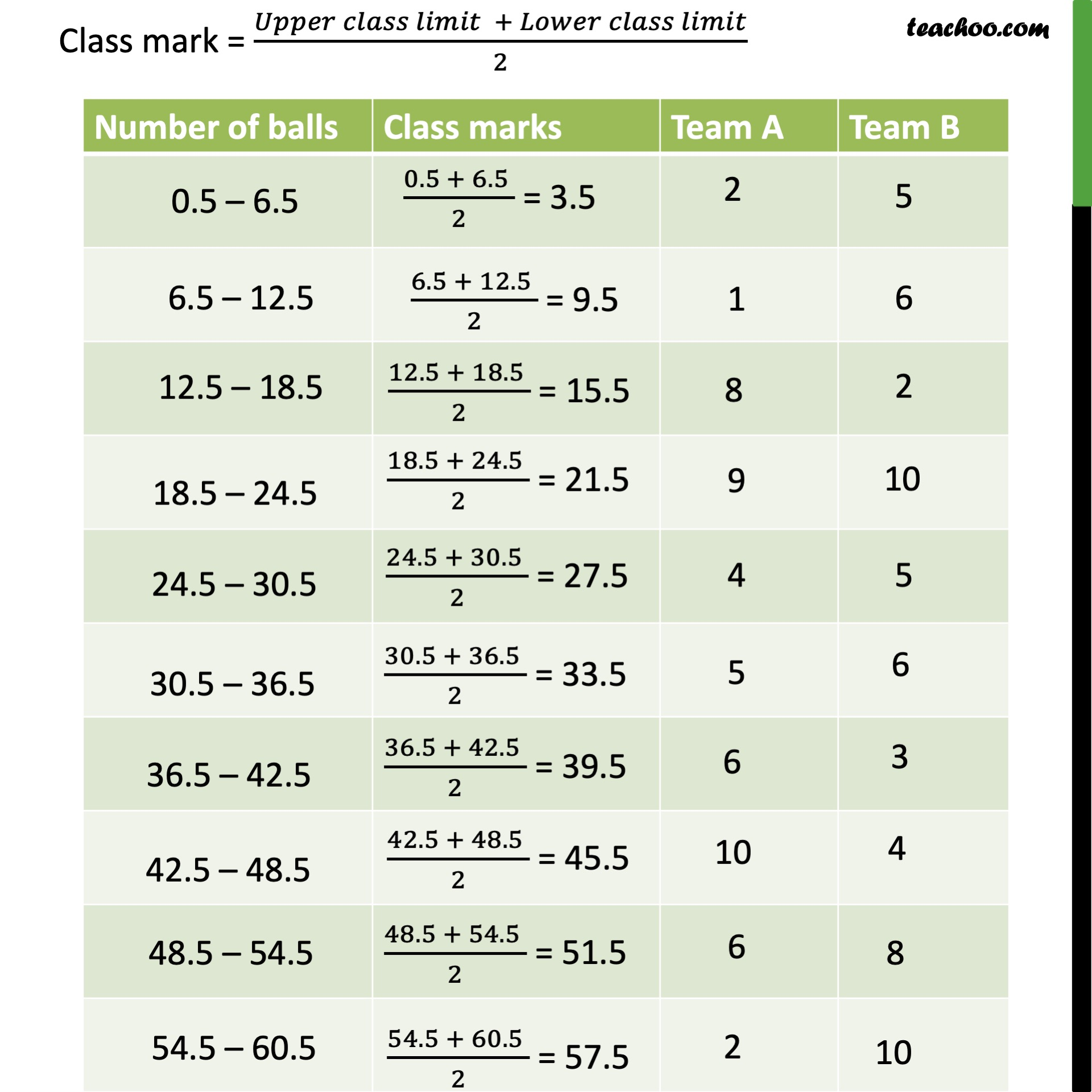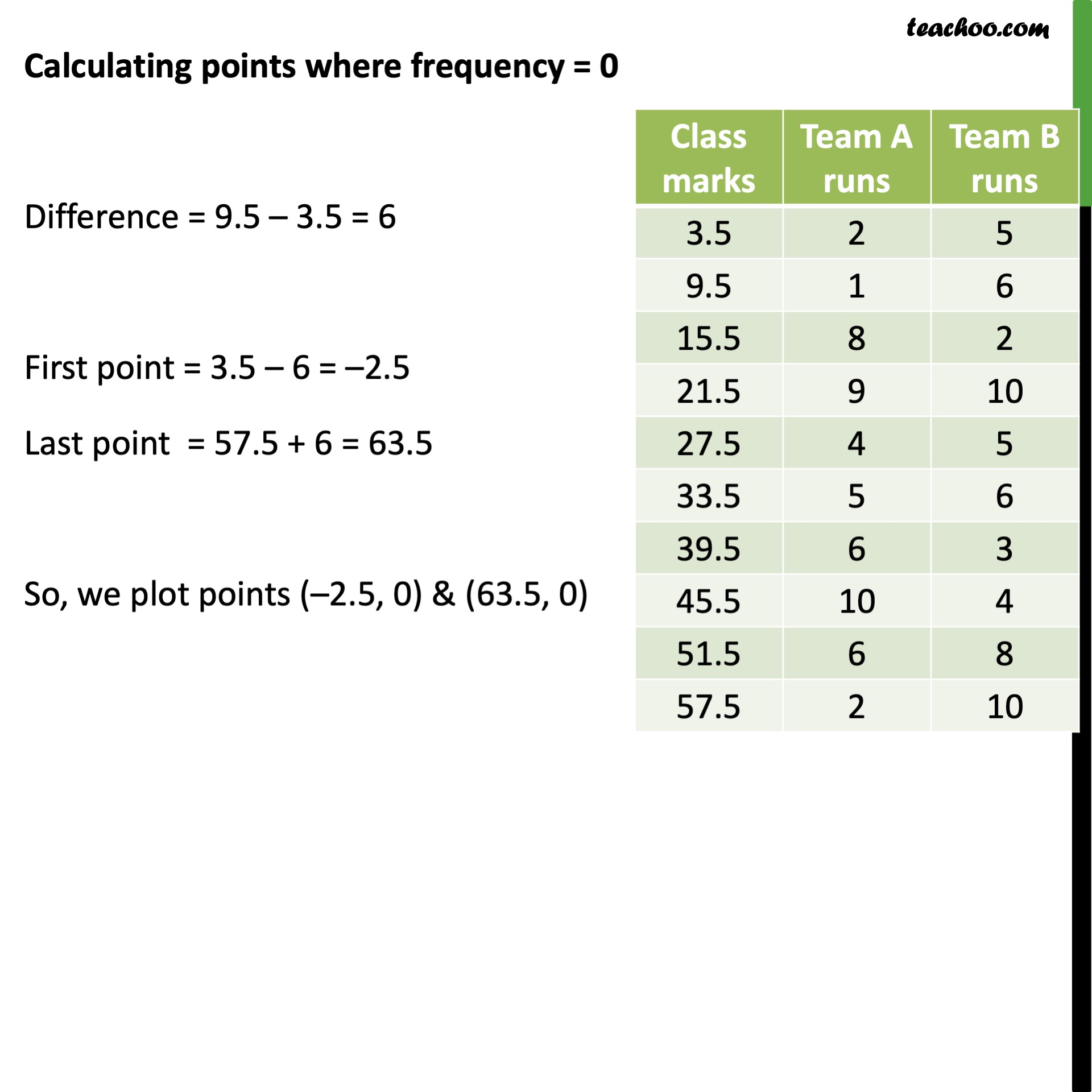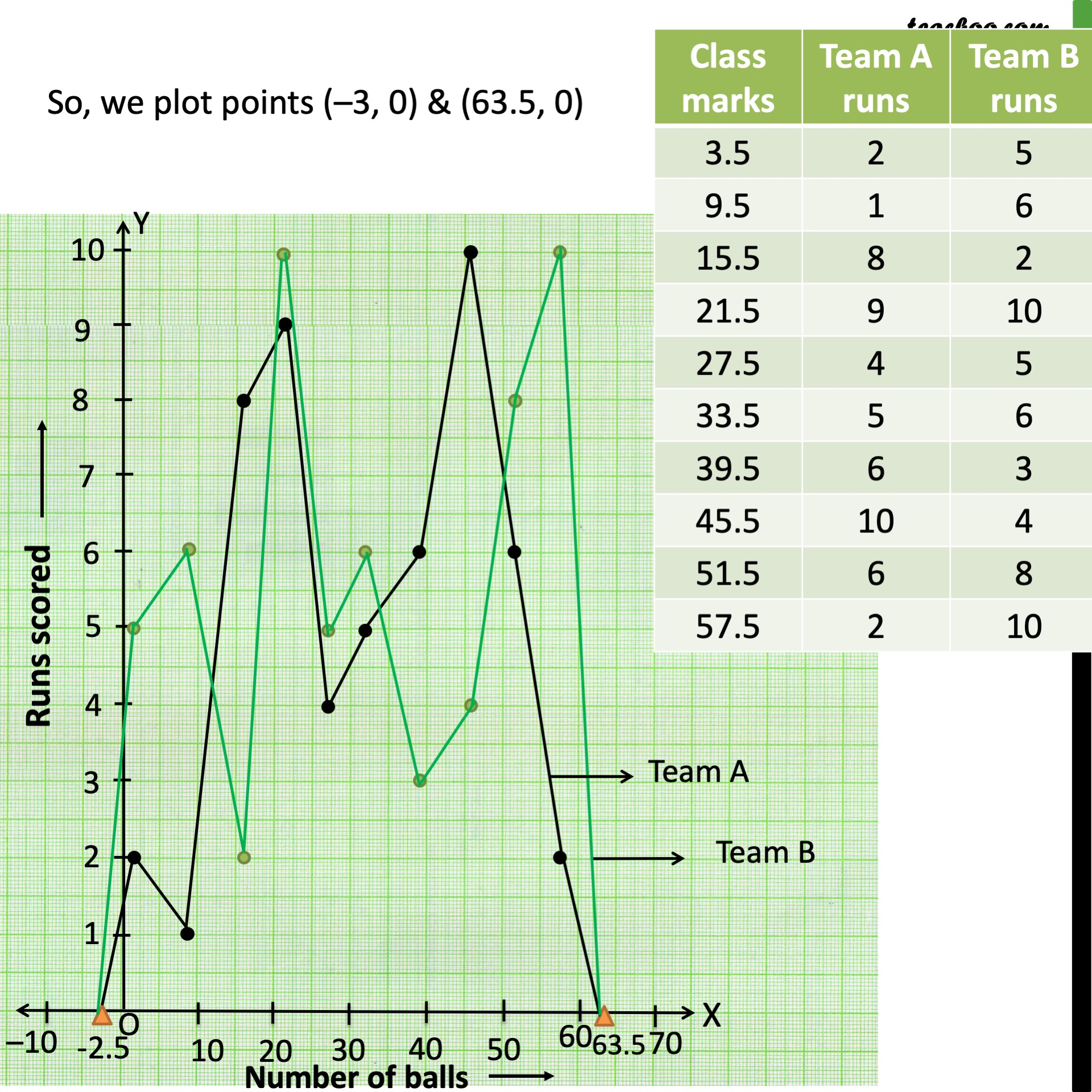Learn in your speed, with individual attention - Teachoo Maths 1-on-1 Class

### Transcript

Ex 12.1, 7 The runs scored by two teams A and B on the first 60 balls in a cricket match are given below: Represent the data of both the teams on the same graph by frequency polygons. [Hint: First make the class intervals continuous.] To draw histogram, we make the data continuous We observe that the gap is 7 – 6 = 1 ∴ 𝐺𝑎𝑝/2 = 1/2 = 0.5 ∴ We add 0.5 to each upper class limit and subtract 0.5 from each lower class limits to make the class intervals continuous.